﻿ Boundary Element Method Applied to the 2D Foil with Oscillating Heave and Pitch MotionsPublications are Open
Access in this journal
Article Versions
Export Article
• Normal Style
• MLA Style
• APA Style
• Chicago Style
Research Article
Open Access Peer-reviewed

### Boundary Element Method Applied to the 2D Foil with Oscillating Heave and Pitch Motions

Mehdi Pourmostafa , Hassan Ghassemi, Parviz Ghadimi
American Journal of Mechanical Engineering. 2020, 8(1), 17-25. DOI: 10.12691/ajme-8-1-3
Received April 13, 2020; Revised May 15, 2020; Accepted May 22, 2020

### Abstract

When an oscillating foil is placed in a uniform and steady flow, due to its heave and pitching motion, it produce a downstream flow at trailing edge, which leads to a forward force or thrust. Many fishes or insects use this phenomenon to move in air/water. It has proved that the heave and pitch frequency is very important parameter to produce thrust which should be chosen sharply. In this paper a 2D airfoil with oscillating movement was simulated and power/thrust coefficient was calculated. An unsteady numerical model based on boundary element method (BEM) was developed to simulate the flow around the foil. Two different conditions (with different angles of attack, α=5, 10) were chosen for the flapping airfoil. For each condition, the foil was oscillated with 8 different frequencies to draw the thrust and power coefficients. The results were compared with experimental data and a finite volume-based solver. It could be seen that however FVM is more precisions to simulate such a hydrodynamics cases but for lifting bodies objects such as airfoil, BEM offers reasonable accuracy. In addition, one of the best advantages of BEM is its speed in calculation. Considering BEM just used 100 boundary cells in simulations, it is about 30 times faster than FVM, which means BEM could be used in rough estimations and preliminary calculations.

### 1. Introduction

Simultaneous heave and pitch movements of an airfoil with forward speed, produce a forwarding thrust. This happens because the oscillating movements of airfoil lead to produce vortexes at top and lower side of airfoil in each moving period. These vortexes (are called Karman Vortex Street) make a jet at the trailing edge and produce forward force. This mechanism firstly proposed by Karman and Burgess 1 in 1935. Afterward a large number of experimental/visualizations, researches were developed to investigate oscillating airfoil (Ohashi & Ishikawa 2 1972; Oshima & Natsume 3 1980; Oshima & Oshima 4 1980). Freymuth 5 (1988), presented his studies on NACA 0012. This airfoil in the wind tunnel was affected by heave and pitch motions. Linear non-viscous theory predicts that with special combination of heave and pitch motion, airfoil efficiency could be increase but experimental visualizations shows less efficiency. In 1968, Schere 6 developed wide range of experiments on an airfoil with moderate high aspect ratio but he never reached efficiency more than 70%. In 1989, Koochesfahani 7 showed that the number of vertices that foil produced in each period is depends on the amplitude and frequency of airfoil. In 1997, Anderson et al. 8 performed an extensive test on an oscillating NACA0012. Her experiments were comprised of visualization and data recording. Visualization was performed at Reynolds of 1100 with PIV method. They also measured thrust/pressure coefficient at Reynolds 40000. At last they showed that with good combination of heave/pitch motion, efficiency better than 85% could be reached. Pedro 9 (2003), simulated a two-dimensional flapping hydrofoil at Reynolds number of 1100. He used ALE method to track the moving boundaries of moving hydro foil. The obtained results showed sensitivity of efficiency and thrust to Strouhal number, pitch and phase angle. Motivated by aerodynamic of insect flight, Wang 10 developed a CFD tools to simulate flow around a two-dimensional moving wing. They focused on frequency selection in forward flapping flight. Only heaving motion was investigated. Developing Wang research in harmonic movement of a foil, Guglielmini et al. 11 added pitching movement to oscillating foil. They tried to compute torque and force due to heave/pitch motion and compared the results to experimental data. Good agreements were showed in their publication. Jung et al. 12 carried out an experimental investigation to study the unsteady characteristics of vortex shedding in the near wake of an oscillating airfoil. The airfoil was harmonically pitched and the velocity in the wake was measured by means of hot wire anemometry. In 2008, Mantia et al. 13 used a new formulation of unsteady Kutta condition to simulate potential flow around a two-dimensional oscillating foil. They calculate hydrodynamic forces on heave/pitching foil and compared the results with experimental data. In 2009, Zhi-Dong et al 14 examined the effect of different locations of the pitching axis of a 2D oscillating flexible foil with FLUENT. They investigated 3 deflexion mode and showed that highest propulsive efficiency is achieved with pivot axis at 1/3 of chord length from leading edge. Zhang et al. 15 investigated 2D flapping foil under influence of vortices which were generated by a D-section cylinder. They used a RANS based software for simulations. Xie et al 16 numerically studied a flapping foil for energy extraction. They showed that power extracted from an oscillating air foil is mainly due to heaving rather than pitching motion. In 2015, Tseng and Cheng 17 presented a numerical model to investigate fluid-structure interaction for a 2D flow over a sinusoid-pitching foil. They compared the numerical results with experimental data and showed the numerical model is sufficient to predict the dynamic stall phenomenon. Zhang et al. 18 studied the vortex shedding and formation behind an isolated cylinder, under the wake generation of an oscillating airfoil. The foil was oscillated with pitch movement and in different frequencies. In 2016, Caja at al. 19 applied a potential flow theory to study an oscillating foil and then a cycloidal propeller. They also studied the effect of added mass on their simulations. In 2017, numerical versus experimental investigation of energy extraction performance of a flapping foil was performed by Xu et al 20. In this study, pitch and heave motion of the airfoil were adjust through a crankshaft–like structure. Comparison between numerical and experimental data showed good agreement. They also showed that higher efficiency can be achieved with larger pitch amplitude at medium frequencies. Iverson et al. 21 experimentally studied the oscillation of a NACA 0015 foil with an aspect ratio of 7.5 in a water tunnel to assess fidelity of model-scale representations of full-scale oscillating-foil turbines. They investigate the effect of dynamic stall, channel confinement and forced transition in Reynolds number range from 20000 to 50000. In 2019, Iverson et al. 22 experimentally studied the effect of structural properties (moment of inertia and stiffness) and kinematic parameters on the propulsive performance of two oscillating foil. They also shown that the trailing edge angle of the foil and the incident flow direction have the highest influence on thrust production. Jiang et al. 23 in 2020, numerically investigated the energy extraction performance of the flow energy convertor based on oscillating (pitch and swing motions) foil. They described a linear equation between power coefficient and foil length. Numerical simulation shows that the loss caused by 3D effect is mainly attributed to the tip leakage flow.

In this paper, a hydrodynamic 2D solver based on BEM is proposed to simulating flow on lifting bodies. This solver is applied to calculate hydrodynamic forces on an oscillating (heaving/pitching) airfoil. Thrust and pressure coefficient is calculated and compared with a 2D FVM solver and experimental data.

### 2. Governing Equations and Numerical Implementation

Inviscid, incompressible and irrotational flow could be expressed in form of 2D Laplacian equation: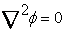(1)

Appling Greens second identity, one can easily find: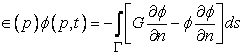(2)

Eq. (2) represents the integral representation of the solution for the Laplace equation (Eq. 1), where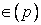is the free term coefficient, which depends on the position of point p and is defined as 24: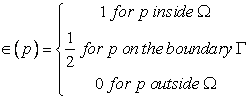(3)

In this study, as we used 2D equations, the Green function is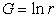and also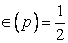assumed all over the boundaries. So, the unsteady form of Laplacian equation (Eq. 2) can be written as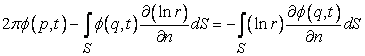(4)

Based on Hess 25 formulation, the Kelvin’s theorem should be satisfied for lifting bodies; so, the integral should be expanded over the wake sheet downstream the trailing edges.

As it is necessity to simulate a moving object, the moving velocity of the body should be considered. Consider v(t) and ω(t) to be linear and angular velocity of the body respectively, so the total velocity of each panel is calculated as follows: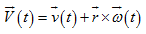(5)

To compute the perturbation velocity over wake sheet, the gradient from equation (4) should be taken as 26: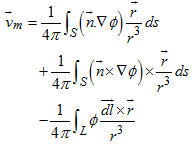(6)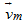is the mean velocity of upper and lower part of the wake sheet.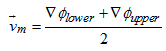(7)

Last term in Eq. (6) is taken over free part of the wake surfaces. These integrands are equivalent to Biot-Savart 27 formula to calculate the induced velocities. Discretized form of Eq. (6) based on the BEM is expressed as follow: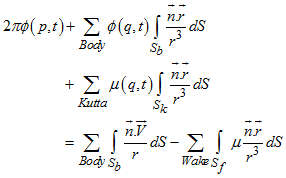(8)

Expanding Eq. (4) over all panels over the body, it is also needed to apply the no flux kinematic boundary condition 28 and Kutta condition at trailing edge, given by Eqs. (9) and (10).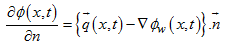(9)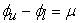(10)

where μ is the intensity of dipoles on the wake panel adjacent to trailing edge.

Delhommeau 29 presented the analytical solution of the source and doublet distribution over the body. His solution is used for discretization of Eq. (8) leads to a system of equations in the form Eq. (11).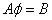(11)

The coefficient matrix can be solved using common iterative methods to calculate the doublet intensity over the body. In this article, the successive over relaxation (SOR) method is utilized. In the next step, it is necessary to compute the perturbation velocity on the free vortex sheet. For this reason, Eq. (6) is solved explicitly on all wake nodes to find the mean velocities. Based on Hess formulation, the Kelvin’s theorem should be satisfied for the lifting bodies. Hence, the integral should be expanded over the wake sheet, downstream of the trailing edges.

Since it is necessary to simulate a moving object, the moving velocity of the body should be considered. Considering the fact that v(t) and ω(t) are linear and angular velocity of the body, respectively, the total velocity of each panel is computed by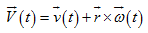(12)

Details of the implemented algorithm can be found in the work of Najafi and Abbaspour 30.

Solving Eq. (11) with a common iterative method like SOR, ϕ would achieve on all panels. Then one can calculate pressure distribution by Bernoulli equation: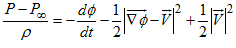(13)

Force and moments can be calculated as follows: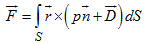(14)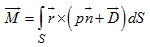(15)

Where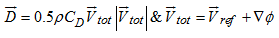(16)

In above equation,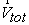is total velocity relative to an observer in moving.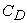is local friction coefficient over the body surface. For a smooth plate in turbulent flow, it is given by 31: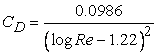(17)

For better understanding the algorithm of the written code is shown in Figure 1.

• Figure 1. Applied algorithm for BEM solver

### 3. Oscillating Foil

NACA0012 is used for oscillating in uniform flow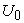and extracting hydrodynamic forces. The foil heaving and pitching motion functions are h(t) and θ(t) respectively (ω is frequency of oscillating motions) (Figure 2). As we can see, pitch motion, has ψ phase angle (respect to heave motion). These equations are as follows 32: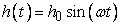(18)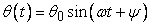(19)

Under these motions, the foil subjected to forces (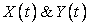in x and y directions) and moment (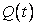about z axis) which one can calculate power and thrust using following formula: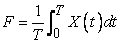(20)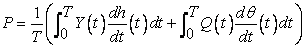(21)

Note that X(t),Y(t) and Q(t) are calculated from Eqs. (14) and (15).

Thrust and power coefficients are defined as: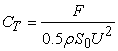(22)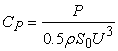(23)

where ρ denote the fluid density and S is the area of one side of foil.

The Strouhal number is defined as follows: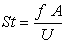(24)

where f is frequency of oscillation and A is the width characteristic of the created jet flow. Since A is unknown before simulation, so Strouhal number defined based on the total excursion (peak to peak) of the trailing edge of the foil (ATE) as follows: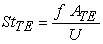(25)

In this paper, two different condition with various angle of attack (α=5&10) is simulated. Reynolds number was set to Re=40000. Distance of the point which the airfoil pitches about, from leading edge is b and the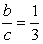. If phase angle between heave and pitch motion is 90° than the angle of attack is: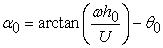(26)
• Figure 2. Geometry of the foil and lift, drag and moment about its pivot point

### 4. Validation

Based on BEM, a computer software was written. To apply the solver to the defined problem, it is needed to discretize the boundary of the air foil. Discretized air foil with 100 cells is showed in Figure 3.

• Figure 3. Discretized 100 cells on the foil

Before simulating the oscillating airfoil, the fixed airfoil was investigated to validate the numerical model in calculating hydrodynamic forces. For this purpose, pressure coefficient for NACA0012 was calculated in two angles of attack (α0=5&10). Pressure coefficient distribution is compared with experimental data (Figure 4 and 5) 33.

• Figure 4. Pressure coefficient distribution for NACA0012 in angle of attack α0=6
• Figure 5. Pressure coefficient distribution for NACA0012 in angle of attack α0=10

Lift coefficient is also compared with experimental data is shown in Figure 6. It can be seen that, the results obtained are very close to experimental data and the numerical solver shows good agreement.

• Figure 6. Lift coefficient for NACA0012 in angle of attack α0=10

After simulating of fixed foil, an oscillating NACA0012 is investigated. The upstream velocity is assumed to be V=1m⁄s and the time step is set as 0.1s. The purpose of this simulation is to validate the moving airfoil hydrodynamic forces, compared to analytical data. The analytical solution is based on a flat plate airfoil which moves by a constant velocity of U, as shown in Figure 7.

• Figure 7. Oscillating pitch and heave motion of a flat plate.

The harmonic heaving and pitching motions are supposed to satisfy Eq. (27) with the same frequency.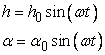(27)

For such assumptions, the lift force is calculated by following formula 27: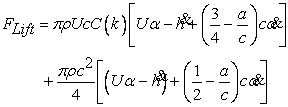(28)

This can be written as circulatory lift L1 in steady motion added by mass lift L2 due to acceleration, as in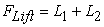. Also, C(k) is the deficiency factor which is plotted versus the reduced frequency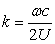, which is presented in Figure 8.

• Figure 8. Lift deficiency versus reduced frequency.

The simulations are conducted for three separate conditions. Pure heaving, pure pitching, and combined heave/pitch motion parameters are displayed in Table 1.

The airfoil can be rotated about the axis that is located at the leading edge. All the simulations were performed up to 25 seconds which are approximately 5 times of the periodic frequency. The lift forces due to heaving, pitching and flapping are plotted in Figure 9. As shown, comparison of the numerical results shows good agreement between the presented model and the analytical solution.

• Figure 9. Lift forces for the oscillating foil

### 5. Numerical Implementation and Results

In this section, the main purpose of this article is investigated. The oscillating airfoil was subjected to flow with unit velocity at two different angles of attack (α0=5&15). For each angle of attack, 8 simulation was performed in different Strouhal numbers. The time step was set to 0.05s. The conditions are shown in Table 2 and Table 3.

For better comparison, the simulations are performed with a FVM based solver 34, 35. The discretized domain for FVM simulation, comprised of 18706 cells, is shown in Figure 10.

• Figure 10. Computational domain for FVS with 18706 cells.

Figure 11 shows the time history of heave and pitch motions for two Strouhal numbers (St = 0.2744 and St=0.2716) in about 11sec real-time simulation.

Time history of lift and drag forces on airfoil was plotted for one sample Strouhal number in each case (Figure 12). As it was expected, drag frequency is twice of the lift frequency for each case. In Figure 12, the blue line denotes the thrust (drag). Thrust is more negative due to x axis (direction of x axis is from leading edge to trailing edge, Figure 2), this means that a propulsion force is acting on the air foil, due to oscillating motions.

• Figure 11. Heave and pitch motions of air foil
• Figure 12. Lift and drag of airfoil

Pressure coefficient was plotted against the chord of airfoil in two time steps in cases 1 & 2. In Figure 13, t=7.20 and t=9.10 was chosen. At t= 7.20, the airfoil is nearly at the top of its harmonic heaving motion and the angle of attack is very close to zero (θ=0.48) (that is why the pressure coefficient is almost symmetry), but the accelerating motion caused pressure distribution on airfoil. At t=9.10, the air foil is at the center of it path on heaving motion, but the angle of attack is almost large (θ=32). That is the reason for high pressure distribution on leading edge of foil.

• Figure 13. Pressure coefficient (St = 0.2744– case 1)
• Figure 14. Pressure coefficient (St = 0.2716 – case 2)

For airfoil in case 2, t=7.05 and t=10.30 was chosen at Strouhal number equal to 0.2716. In these two times, the foil is at the top (t=7.05) and bottom (t=10.30) of the heaving path (Figure 14). The angle of attack is θ=10.5 & θ=22.3 respectively.

After each simulation, thrust and power coefficients was calculated from Eqs. (22-23). Figure 15-18 show comparison between numerical results and experimental data.

• Figure 15. Thrust coefficient for case 1
• Figure 16. Power coefficient for case 1
• Figure 17. Thrust coefficient for case 2
• Figure 18. Power coefficient for case 2

All simulations were carried out with a core i5 @ 2.5 GHz CPU. Each time step for FVM solver was last about 3 sec while it was 0.1 sec for BEM. That is because of the discretized cells in the computational domain. FVM was solved for 18706 cells while BEM could simulate the case with only 100 cells. This leads to a massive reduction in simulation time. Both solvers show reasonable accuracy, but as it was expected, FVM is a little more accurate than BEM solver. This is most probably because of viscosity affects which is applied virtually in BEM solver; so, it is recommended to use BEM in preliminary designs and primary estimations.

### 6. Conclusion

Simulation of an oscillating airfoil in the uniform flow was the main calculation results for this paper. To simulating such a lifting problem, BEM was chosen because of its powerful ability to simulate lifting bodies. Potential velocity in this solver is a simplified form of Navier-Stokes equations. Air foil was simulated in two different angles of attack (α0=5&15). Thrust and power coefficient was calculated and compared with finite volume solver and experimental data. In low Strouhal number, proposed solver showed great agreement related to experimental data, but whatever the frequency of oscillating goes higher, the error appears in numerical results and that is because of complexity of flow in high oscillating of airfoil. In this region, the flow is more three-dimensional. In this solver the flow is set to two-dimensional equation in the manner of comparison with experimental data, however it is claimed that the experimental data was performed two dimensionally but as we know in reality it is very hard (or sometimes impossible) to test the airfoil two dimensionally.

Another interesting parameter in these simulations is computational time; it is a parameter which plays and important role to choose the computational method, especially in rough estimations. Each time step in FVM last about 3 second. That is because of the fractional steps algorithm. Convection, diffusion and pressure Poisson equations solved iteratively in each time steps. However, the corresponding time in BEM is 0.1 sec. another reason for such a big difference is the computational domain. As shown in previous sections, the computational domain for FVM required 18706 cells while the BEM just needed 100 boundary cells. So, for rough estimation or predictions, it is suggested to use BEM at the beginning and use FVM where more precision calculation required.

### References

  Karman, T., Burgess, J. M, "General aerodynamic theory of perfect fluid", Vol II, Leipzig: Springer Verlag, p.308, 1935. In article  Ohashi, H., Ishikawa, N., "Visualization study of a flow near the trailing edge of an oscillating airfoil", Bulletin of the Japanese Society of Mechanical Engineers, 15:840-845, 1972. In article View Article  Oshima, Y., Natsume, A., "Flow field around an oscillating airfoil", In Flow Visualization II. Proceedings of the Second International Symposium on Flow Visualization, Bochum, W. Germany (ed. W. Merzkirch), 1980, pp. 295-299. In article  Oshima, Y., Oshima, K., "Vortical flow behind an oscillating airfoil", In Proceedings of the 15th International Congress, International Union of Theoretical and applied Mechanics, 1980, 357-368. Amesterdam: North Holland Publishing Co. In article  Freymuth, P., "Propulsive vertical signature of plunging and pitching airfoils", AIAA Journal, 1988, 26:881-883. In article View Article  Schere, J. O., "Experimental and theoretical investigation of large amplitude oscillating foil propulsion systems", US Army Engineering Research and Development Laboratories, 1968. In article  Koochesfahani, M., "Vortical patterns in the wake of an oscillating airfoil", AIAA Journal 27, 1989, 1200-1205. In article View Article  Anderson, J. M, Streitlien, K., Barrett, D. S., Triantafyllou, M. S., "Oscillating foils of high propulsive efficiency", J. Fluid Mech. Vol., 1977, 360:41-72. In article View Article  Pedro, G., Suleman, A., Djilali, N., "A numerical study of the propulsive efficiency of a flapping hydrofoil", International journal for numerical methods in fluids, 2003, 42:493-526. In article View Article  Wang, J., "Vortex shedding and frequency selection in flapping", flight. J. Fluid Mech, 2000, Vol. 410:323-341. In article View Article  Guglielmini, L., Blondeaux, P., "Propulsive efficiency of oscillating foils", European Journal of Mechanics B/Fluids, 2004, 23: 255-278. In article View Article  Jung, Y. W, Park, S. O, "Vortex-shedding characteristics in the wake of an oscillating airfoil at low Reynolds number", Journal of Fluid and Structures, 2005, 20:451-461. In article View Article  Mantia, M. L, Dabnichki, P., "Unsteady panel method for flapping foil", Engineering analysis with boundary elements, 2008, 33:572-580. In article View Article  Zhi-dong, W., Wen-chao, C., Xiao-qing, Z., "Propulsive performance and flow field characteristics of a 2-D flexible fin with variations in the location of its pitching axis", J. Marine. Sci. Appl, 2009, 8:298-304. In article View Article  Zhang, X., Su, Y., Yang, L., Wang, Z., "Hydrodynamic Performance of flapping-foil propulsion in the influence of vortices", J. Marine. Sci. App, 2010, 9:213-219. In article View Article  Xie, Y., Lu, K., Zhang, D., "Investigation on energy extraction performance of an oscillating foil with modified flapping motion", Renewable Energy, 2013, 63:550-557. In article View Article  Tseng, C. C, Cheng, Y. E, "Numerical investigations of the vortex interactions for a flow over a pitching foil at different stages", Journal of fluids and structures, 2015, 58:291-318. In article View Article  Zhang, G. Q, Ji L. C., "Investigation of two degrees of freedom on vortex-induced vibration under the wake interference of an oscillating airfoil", Acta Astronautica, 2016. In article View Article  Sanchez-Caja, A., Martio, J., "On the optimum performance of oscillating foil propulsors", J Mar Sci Technol, 2016, 22:114-124. In article View Article  Xu, G. D., Xu, W. H., Dai, J., "Numerical and experimental study of a flapping foil generator", Applied ocean research, 2017, 63: 242-250. In article View Article  Iverson, D., Boudreau, M., Dumas, G., Oshkai, P., "Boundary layer tripping on moderate Reynols number oscillating foils", journal of fluid and structures, 2019, 89, 1-12. In article View Article  Iverson, D., Rahimpour, M., Lee, W., Kiata, T., Oshkai, P., "Effect of chordwise flexibility on propulsive performance of high inertia oscillating-foils", Journal of fluids and structures, 2019, 91. In article View Article  Jiang, W., Wang, Y.L., Zhang, D., Xie, Y.H., "Numerical investigation into the energy extraction characteristics of 3D self-induced oscillating foil", Renewable energy, 2020, 148: 60-71. In article View Article  Katsikadelis, J. T, "The boundary element method for engineers and scientists", Elsevier. Second edition, 2016. In article View Article  Hess, J. L., "Calculation of potential flow around arbitrary three-dimensional lifting bodies", McDonnell Douglas Corporation, 1972, Report no. MDCJ 5679-01. In article View Article  Politis, G. K, "Simulation of unsteady motion of a propeller in a fluid including free wake modeling", Elsevier Engineering Analysis with Boundary Elements, 2004, 28: 633-653. In article View Article  Katz, J., Plotkin, A., "Low-Speed Aerodynamics", Singapore. McGraw-Hill, 1991. In article  Politis, G. K., "Application of a BEM time stepping algorithm in understanding complex unsteady propulsion hydrodynamic phenomena", Elsevier, Ocean Engineering, 2011, 38: 699-711. In article View Article  Delhommeau, G., "Les Problemes de Diffraction-Radiation et de Resistance de Vagues: Etude Theorique et Resolution Numerique par la Methode des Singularites", These d'Etat, 1988, E.N.S.M. Nantes, France. In article  Najafi, S., Abbaspoor, M., "Numerical investigation of flow pattern and hydrodynamic forces of submerged marine propellers using unsteady boundary element method", Proceedings of the Institution of Mechanical Engineers, 2017, Part M: Journal of Engineering for the Maritime Environment, vol. 232, pp. 1-13 (ISSN: 14750902). In article View Article  Blevins, R. D, "Applied fluid dynamics handbook", Princeton, NJ: Van Nostrand Reinhold Company, 1984. In article  Abbasi A., Ghassemi, H, “Hydrodynamic performance of the 3d hydrofoil at the coupled oscillating heave and pitch motions”, to be appear in Cogent engineering, 2020. In article  Gregory, N., O’reilly, C., "Low-speed aerodynamic characteristics of NACA 0012 aerofoil section, including the effects of upper-surface roughness simulating hoarfrost", National Physical Laboratory Teddington, England, 1970. In article  Pourmostafa, M., Seif, M.S., "Multigrid methods in free surface flow simulations", 13th Numerical Towing Tank Symposium (NUTTS 10), 2010. In article  Heidarian, A.R., Ghassemi, H, Liu P., “Numerical aerodynamic of the rectangular wing concerning to ground effect”, American Journal of Mechanical Engineering. 2018, 6(2), 43-47. In articleThis work is licensed under a Creative Commons Attribution 4.0 International License. To view a copy of this license, visit http://creativecommons.org/licenses/by/4.0/